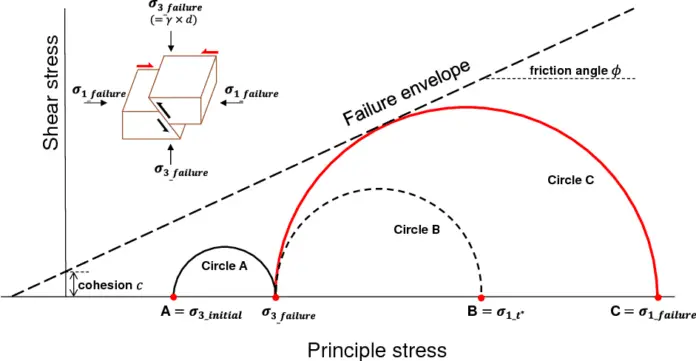# Angle of Internal Friction | Angle of Shearing ResistanceOne of the important parameters regarded as a typical property of granular soils is the angle of internal friction, ϕ. The ability of a rock or soil mass to withstand shear stress can be measured by the angle of internal friction (also called the angle of shearing resistance). When failure occurs in response to a shearing stress (τ), the angle (ϕ), measured between the normal force (σ) and resultant force (R), is called the angle of internal friction.

The coefficient of sliding friction is its tangent (τ/σ). The angle of internal friction of any soil can be seen visually on a Mohr’s circle plot after the shear strength test.

Experimental analysis such as the triaxial test is used to determine the angle of internal friction’s value. Prior to engaging in analytical and design processes in relation to foundations, retaining walls, slope stability, and earth-retaining structures, shear strength parameters must be determined. A physical characteristic of earth materials, or the slope of a linear representation of their shear strength, is the angle of internal friction.

The internal resistance a soil mass can provide per unit area to withstand failure along any internal plane is known as shear strength. Failure happens when this resistance is exceeded. The maximum or limiting value of shear stress created within a soil’s matrix prior to yielding is referred to as the soil’s shear strength. The cohesive and frictional forces between adjacent particles in a soil matrix are what give the structure its shear strength.

As a result, there is some surface dependence on the soil shear strength. Any action that prevents or encourages soil particle interlocking or welding will inevitably affect soil shear strength.

Shear strength typically consists of:

(a) internal friction or the resistance due to the interlocking of the particles, represented by an angle, ϕ;
(b) cohesion or resistance due to the forces tending to hold the particles together in a solid mass. The cohesion of soil is generally symbolized by the letter ‘c’.

Coulomb was the first to propose the law governing the shear failure of soils, which is represented by the equation;

τ = c + σtanϕ ——— (1)

where the normal force is σ and the shear strength is τ.

Engineers and geologists commonly refer to unconsolidated and uncemented earth materials as soil, while geologists may refer to such materials as sediment. A variety of sizes (mm to m) from very fine to very coarse minerals or rock fragments make up soil (clay, silt, sand, gravel, cobble, and boulder-size).

A mass of grains that are chemically and mechanically distinct from one another can be relatively easily mined, and the removed material can be piled up in a conical shape with slopes known as the angle of repose (Figure 2). The angle of repose is a depiction of the angle of internal friction, but it is typically controlled by grain form, resulting in slopes that are typically between 28° and 34° for piles of loose, dry grains in natural soil.

The angle of internal friction is defined as the angle between the normal reaction force and the combined force of friction and normal reaction force as the object begins to move, whereas the angle of repose is defined as the minimum angle of an inclined plane that causes an object to slide down the plane. Theoretically, the angle of internal friction and the angle of repose appears to mean the same thing. However, the angle of internal friction determined during testing is used for geotechnical designs.

The distribution of grain size, angularity, and particle interlocking are the main variables that affect a soil’s friction angle in addition to density. As you may anticipate, fine-grained and well-rounded sand has a lower friction angle than angular and coarse sand.

φ = 45° Select, granular soil. Slope, φ, maybe even greater if the soil is well compacted.
φ = 30° Good soil. The soil may be uncompacted, or possibly moist.
φ = 15° Poor soil. Poor soil may contain a high percentage of fines and may be wet.
φ = 0° Mud. The soil is liquid, and has no slope angle, φ

## The angle of internal friction of different types of soils

The angle of internal friction for different types of soil can be estimated from the in-situ geotechnical engineering test. Some of them are shown in Table 1;

The angle of internal friction for different soil classifications is provided in Table 2;

#### 1 COMMENT

1.Samir Radi

Good article and helpful. Not mentioned any sources.
Actually, I’m trying to find which ASTM or BS applies for this test procedures.